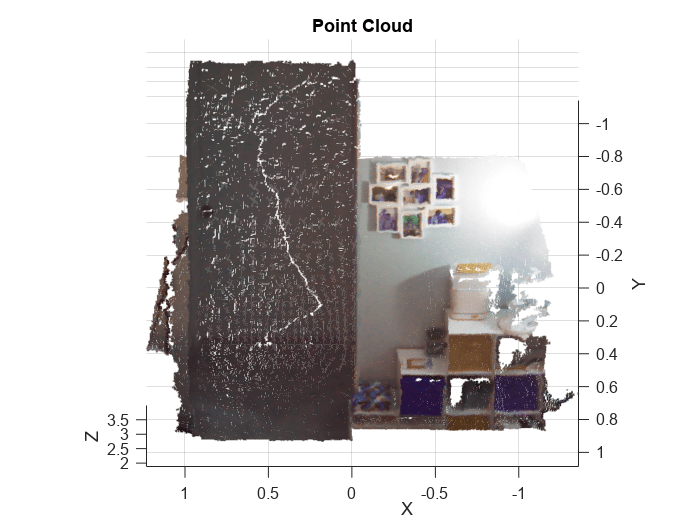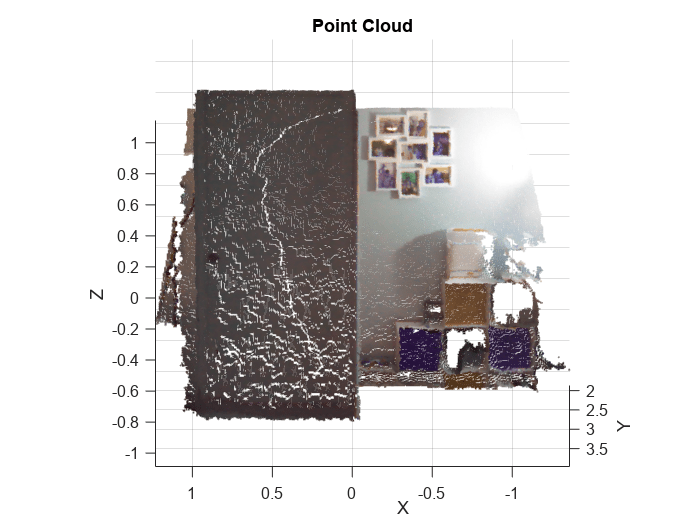# rosApplyTransform

Transform ROS and ROS 2 message entities into target frame

Since R2021a

## Syntax

``tfentity = rosApplyTransform(tfmsg,entity)``

## Description

example

````tfentity = rosApplyTransform(tfmsg,entity)` applies the transformation represented by the `'TransformStamped'` ROS or ROS 2 message to the input message structure `entity`. This function determines the message type of `entity` and applies the appropriate transformation method to it.```

## Examples

collapse all

Load ROS 2 point cloud and transformation messages.

`load("ros2PtcloudMsgs.mat")`

Visualize the point cloud message `ptcloudMsg1`.

```rosPlot(ptcloudMsg1) xlabel('X') ylabel('Y') zlabel('Z') view([-180 80])```Note that the vertical direction in the point cloud is represented by the y-axis. You can transform the point cloud such that the z-axis represents the vertical direction. To accomplish this transformation, use the `geometry_msgs/TransformStamped` message, `tfMsg`, which contains a transform that rotates the points to a target frame that is `90` degrees in the clockwise direction along x-axis. Read the translation and rotation values from `tfMsg` using the `rosReadTransform` function.

`[trans,rot] = rosReadTransform(tfMsg,OutputOption="pair")`
```trans = 3×1 0 0 0 ```
```rot = 3×3 1.0000 0 0 0 0.0000 1.0000 0 -1.0000 0.0000 ```

Transform the point cloud to the target frame using the `rosApplyTransform` function.

`transformedPtcloudMsg = rosApplyTransform(tfMsg,ptcloudMsg1);`

Visualize the transformed point cloud. Note that the vertical direction is now represented by the z-axis.

```rosPlot(transformedPtcloudMsg) view(-180,14)```## Input Arguments

collapse all

Transformation message, specified as a `TransformStamped` ROS or ROS 2 message handle. The `tfmsg` is a ROS or ROS 2 message of type: `'geometry_msgs/TransformStamped'`.

ROS or ROS 2 message, specified as a message structure.

Supported messages are:

• `geometry_msgs/PointStamped`

• `sensor_msgs/PointCloud2`

• `geometry_msgs/PoseStamped`

• `geometry_msgs/QuaternionStamped`

• `geometry_msgs/Vector3Stamped`

## Output Arguments

collapse all

Transformed ROS or ROS 2 message, returned as a message structure.

## Version History

Introduced in R2021a